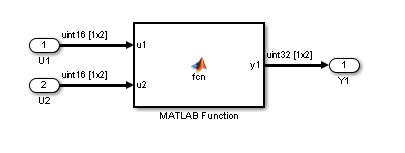## MATLAB Functions

### himl_0001: Usage of standardized MATLAB function headers

ID: Titlehiml_0001: Usage of standardized MATLAB® function headers
Description

When using MATLAB functions, use a standardized header to provide information about the purpose and use of the function.

Rationale

A standardized header improves the readability and documentation of MATLAB functions. The header should provide a function description and usage information.

• By Task > Modeling Standards for DO-178C/DO-331 > High-Integrity Systems > MATLAB > Check usage of standardized MATLAB function headers

• By Task > Modeling Standards for IEC 61508 > High-Integrity Systems > MATLAB > Check usage of standardized MATLAB function headers

• By Task > Modeling Standards for IEC 62304 > High-Integrity Systems > MATLAB > Check usage of standardized MATLAB function headers

• By Task > Modeling Standards for ISO 26262 > High-Integrity Systems > MATLAB > Check usage of standardized MATLAB function headers

• By Task > Modeling Standards for EN 50128 > High-Integrity Systems > MATLAB > Check usage of standardized MATLAB function headers

For check details, see Check usage of standardized MATLAB function headers (Simulink Check).

References

DO-331, Section MB.6.3.4.e – Source code is traceable to low-level requirements

Last ChangedR2018b
Examples

A typical standardized function header includes:

• Function name

• Description

• Inputs and outputs (if possible, include size and type)

• Assumptions and limitations

• Revision history

Example:

```% FUNCTION NAME: % avg % % DESCRIPTION: % Compute the average of three inputs % % INPUT: % in1 - (double) Input one % in2 - (double) Input two % in3 - (double) Input three % % OUTPUT: % out - (double) Calculated average of the three inputs % % ASSUMPTIONS AND LIMITATIONS: % None % % REVISION HISTORY: % 05/02/2018 - mmyers % * Initial implementation % ```

### himl_0002: Strong data typing at MATLAB function boundaries

ID: Titlehiml_0002: Strong data typing at MATLAB function boundaries
Description

To support strong data typing at the interfaces of MATLAB functions, explicitly define the interface for input signals, output signals, and parameters, by setting:

• Complexity

• Type

Rationale

Defined interfaces:

• Allow consistency checking of interfaces.

• Prevent unintended generation of different functions for different input and output types.

• Simplify testing of functions by limiting the number of test cases.

• By Task > Modeling Standards for DO-178C/DO-331 > High-Integrity Systems > MATLAB > Check for MATLAB Function interfaces with inherited properties

• By Task > Modeling Standards for IEC 61508 > High-Integrity Systems > MATLAB > Check for MATLAB Function interfaces with inherited properties

• By Task > Modeling Standards for IEC 62304 > High-Integrity Systems > MATLAB > Check for MATLAB Function interfaces with inherited properties

• By Task > Modeling Standards for ISO 26262 > High-Integrity Systems > MATLAB > Check for MATLAB Function interfaces with inherited properties

• By Task > Modeling Standards for EN 50128 > High-Integrity Systems > MATLAB > Check for MATLAB Function interfaces with inherited properties

For check details, see Check for MATLAB Function interfaces with inherited properties (Simulink Check).

References
• IEC 61508-3, Table B.9 (6) - Fully defined interface

• IEC 62304, 5.5.3 - Software Unit acceptance criteria

• ISO 26262-6, Table 1 (1c) - Enforcement of strong typing
ISO 26262-6, Table 1 (1f) - Use of unambiguous graphical representation

• EN 50128, Table A.1 (11) - Software Interface Specifications

• DO-331, Section MB.6.3.2.b - Low-level requirements are accurate and consistent

Last ChangedR2016a
Examples

Recommended:

In the Ports and Data Manager, specify the complexity and type of input `u1` as follows:

• Complexity to `Off`

• Type to `uint16`Not Recommended:

In the Ports and Data Manager, do not specify the complexity and type of input `u1` as follows:

• Complexity to `Inherited`

• Type to ```Inherit: Same as Simulink```.

### Note

To access the Ports and Data Manager, from the toolbar of the MATLAB Function Block Editor, select Edit Data.

### himl_0003: Limitation of MATLAB function complexity

ID: Titlehiml_0003: Limitation of MATLAB function complexity
Description

When using MATLAB functions, limit the size and complexity of MATLAB code. The size and complexity of MATLAB functions is characterized by:

• Lines of code

• Nested function levels

• Cyclomatic complexity

• Density of comments (ratio of comment lines to lines of code)

Note

Size and complexity limits can vary across projects. Typical limits might be as described in this table:

MetricLimit
Lines of code60 per MATLAB function
Nested function levels31,2
Cyclomatic complexity15
Density of comments0.2 comment lines per line of code

1Pure Wrappers to external functions are not counted as separate levels.

2Standard MATLAB library functions do not count as separate levels.

Rationale

• Comprehension

• Traceability

• Maintainability

• Testability

• By Task > Modeling Standards for DO-178C/DO-331 > High-Integrity Systems > MATLAB > Check MATLAB Function metrics

• By Task > Modeling Standards for IEC 61508 > High-Integrity Systems > MATLAB > Check MATLAB Function metrics

• By Task > Modeling Standards for IEC 62304 > High-Integrity Systems > MATLAB > Check MATLAB Function metrics

• By Task > Modeling Standards for ISO 26262 > High-Integrity Systems > MATLAB > Check MATLAB Function metrics

• By Task > Modeling Standards for EN 50128 > High-Integrity Systems > MATLAB > Check MATLAB Function metrics

For check details, see Check MATLAB Function metrics (Simulink Check).

References
• IEC 61508-3, Table B.9 (6) - Fully defined interface

• IEC 62304, 5.5.3 - Software Unit acceptance criteria

• ISO 26262-6, Table 1 (1a) - Enforcement of low complexity
ISO 26262-6, Table 1 (1f) - Use of unambiguous graphical representation

• EN 50128, Table A.1(11) - Software Interface Specifications

• DO-331, Sections MB.6.3.1.e - High-level requirements conform to standards
DO-331, Sections MB.6.3.2.e - Low-level requirements conform to standards